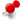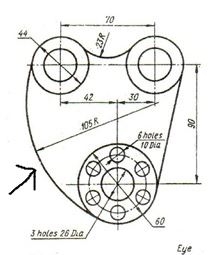# drawing a radius tangent to two circlesClassicListThreaded7 messagesOpen this post in threaded view
|

## drawing a radius tangent to two circlesHi guys just getting into Librecad and would like to know the easiest way to draw the radius of 105 with an arrow pointing to it in the pic thanks
Open this post in threaded view
|

## Re: drawing a radius tangent to two circles

 If you really mean a radius tangent to two circles, it's Circle - Tangential Two Circles, Radius. Cut open the r 105 circle at the tangent points (snap intersection on) and delete the unwanted part. But in the picture it looks like the 105 radius instead intersects with the upper left circle at it's left quadrant. Something different, I don't know a solution for that.
Open this post in threaded view
|

## Re: drawing a radius tangent to two circles

 Hi Dellus, thanks for the reply, I've tried tangential 2 circles radius and cant seem to get it to work, it allows me to pick the first circle. but when I click on the other circle it doesn't recognize it.
Open this post in threaded view
|

## Re: drawing a radius tangent to two circles

 Probably the radius, which is set in the tool options bar above the drawing area, is too small to make a tangent.
Open this post in threaded view
|

## Re: drawing a radius tangent to two circles

 Brilliant Dellus thanks, it worked, now as to the problem with quadrant intersection, I will be sending 2d drawings for the production of templates for tooling, any idea of the margin of error if it doesn't intersect precisely with the quadrant, thanks again